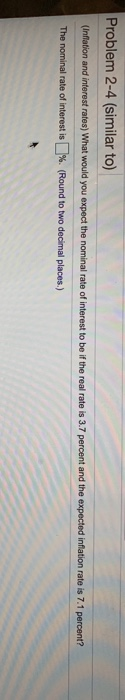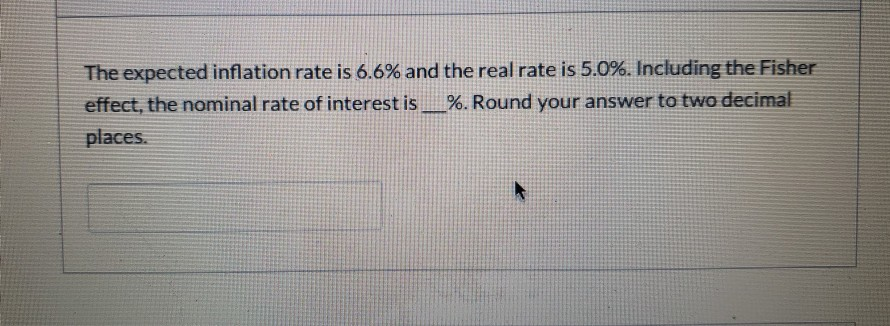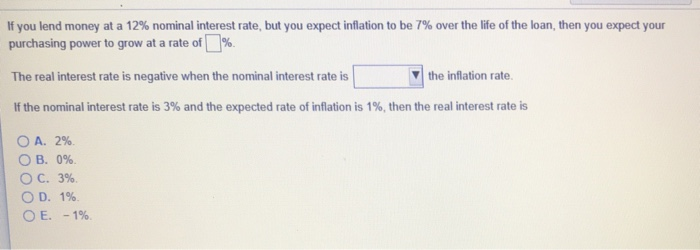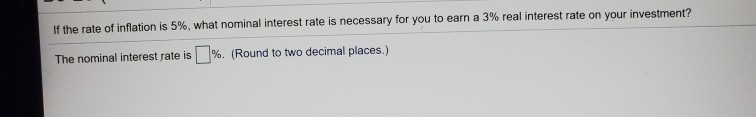Question

# What would you expect the nominal rate of interest to be if the real rate is...

What would you expect the nominal rate of interest to be if the real rate is 5.0 percent and the expected inflation rate is 3.0 ​percent? The nominal rate of interest would be nothing​%. ​(Round to two decimal​ places.)

HI,

Here real rate r = 5%

inflation i = 3%

nominal rate R=?

as per fisher equation

(1+R) = (1+i)(1+r)

1+R = (1+3%)(1+5%)

1+R = 1.03*1.05

R = 8.15%

Hence nominal rate is 8.15%

Thanks

#### Earn Coins

Coins can be redeemed for fabulous gifts.

Similar Homework Help Questions
• ### Problem 2-4 (similar to) (Inflation and interest rates) What would you expect the nominal rate of...Problem 2-4 (similar to) (Inflation and interest rates) What would you expect the nominal rate of interest to be if the real rate is 3.7 percent and the expected inflation rate is 7.1 percent? The nominal rate of interest is %. (Round to two decimal places.)

• ### What would you expect the nominal rate of interest to be if the real rate is...

What would you expect the nominal rate of interest to be if the real rate is 4.2 percent and the expected inflation rate is 6.9 ​percent?

• ### What would you expect the nominal rate of interest to be if the real rate is...

What would you expect the nominal rate of interest to be if the real rate is 4.2 percent and the expected inflation rate is 7.3 percent?

• ### ​(Related to Checkpoint​ 9.6)  ​(Inflation and interest rates​) What would you expect the nominal rate of...

​(Related to Checkpoint​ 9.6)  ​(Inflation and interest rates​) What would you expect the nominal rate of interest to be if the real rate is 4.5 percent and the expected inflation rate is 7.3 ​percent?

• ### nominal rate of interest The expected inflation rate is 6.6% and the real rate is 5.0%. Including the Fisher effect,...nominal rate of interest The expected inflation rate is 6.6% and the real rate is 5.0%. Including the Fisher effect, the nominal rate of interest is __%. Round your answer to two decimal places.

• ### What is the real interest rate if the nominal interest rate is 7​% and the expected...

What is the real interest rate if the nominal interest rate is 7​% and the expected inflation rate is 5% over the course of a​ year? ir = ​(Round your response to two decimal​ places.)

• ### You lent \$370 to a friend for one year at a nominal rate of interest of...

You lent \$370 to a friend for one year at a nominal rate of interest of 3 percent. Inflation during that year was 2 percent. Did you experience an increase or decrease in the purchasing power of your money? How much did it increase or decrease? (Round answer to 2 decimal places, e.g. 52.75%.) The purchasing power ______(increasing or decreasing) by ____%. If the nominal rate of interest is 4.19 percent and the expected rate of inflation is 1.76 percent,...

• ### If the nominal rate of interest is 13.94 %13.94% and the real rate of interest is...

If the nominal rate of interest is 13.94 %13.94% and the real rate of interest is 7.3 %7.3%​, what is the expected rate of​ inflation? The expected rate of inflation is nothing​%

• ### If you lend money at a 12% nominal interest rate, but you expect inflation to be...If you lend money at a 12% nominal interest rate, but you expect inflation to be 7% over the life of the loan, then you expect your purchasing power to grow at a rate of [1%. The real interest rate is negative when the nominal interest rate is If the nominal interest rate is 3% and the expected rate of inflation is 1%, then the real interest rate is ▼| the inflation rate. A. 2%. O B. 096. 3%. 1%....

• ### If the rate of inflation is 5%, what nominal interest rate is necessary for you to...If the rate of inflation is 5%, what nominal interest rate is necessary for you to earn a 3% real interest rate on your investment? The nominal interest rate is %. (Round to two decimal places.)